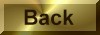Practice Problem 1

Determine the oxidation number of each element in the following compounds:

(a) BaO2     (b) (NH4)2MoO4      (c)Na3Co(NO2)6      (d) CS2

Solution

(a) If the oxidation number of the oxygen in BaO2 were -2, the oxidation number of the barium would have to be +4.  But elements in Group IIA can't form +4 ions.  This compound must be barium peroxide, [Ba2+][O22-].   Barium therefore is +2 and oxygen is -1.

(b) (NH4)2MoO4 contains the NH4+ ion, in which hydrogen is +1 and nitrogen is -3.  Because there are two NH4+ ions, the other half of the compound must be an MoO42- ion, in which molybdenum is -6 and oxygen is -2.

(c) Sodium is in the +1 oxidation state in all of its compounds.  This compound therefore contains the Co(NO2)63- complex ion.  This complex ion contains six NO2- ions in which the oxidation number of nitrogen is +3 and oxygen is -2.  The oxidation state of the cobalt atom is therefore +3.

(d) The most elecronegative element in a compound always has a negative oxidation number.  Since sulfur tends to form -2 ions, the oxidation number of the sulfur in CS2, is -2 and the carbon is +4.